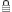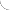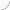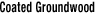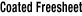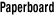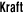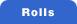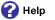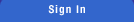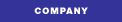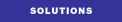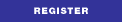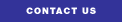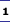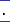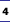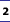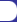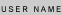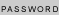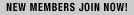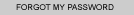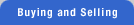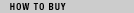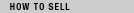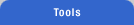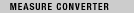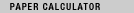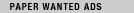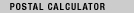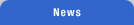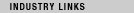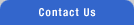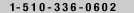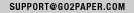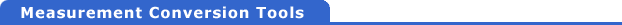Basis Weights  Total Weight  Length  Price  Total Cost  Basis Weight Chart
Basis Weights
 Book Weight Basis Size: 25x38 1 Pounds (lbs) = 1.4802 Grams Per Square Meter (GSM) 1 Grams Per Square Meter (GSM) = 0.6756 Pounds (lbs) Pounds (lbs) GSM GSM Pounds (lbs)
 Cover Weight Basis Size 20 x 26 1 Pounds (lbs) = 2.7042 Grams Per Square Meter (GSM) 1 Grams Per Square Meter (GSM) = 0.3698 Pounds (lbs) Pounds (lbs) GSM GSM Pounds (lbs)
 Newsprint Basis Size 24 x36 1 Pounds (lbs) = 1.6267 Grams Per Square Meter (GSM) 1 Grams Per Square Meter (GSM) = 0.6147 Pounds (lbs) Pounds (lbs) GSM GSM Pounds (lbs)

Basis Weights  Total Weight  Length  Price  Total Cost  Basis Weight Chart
Total Weight
 1 Short Ton = 0.9072 Metric Ton 1 Short Ton = 2000 Pounds (lbs.) 1 Metric Ton = 1.1023 Short Ton 1 Metric Ton = 2,204.6 Pounds (lbs) 1 Pounds (lbs) = 0.0005 Tons 1 Pounds (lbs) = 0.0004536 Metric Tons 1 Pounds (lbs) = 0.4536 Kilograms (kg) 1 Pounds (lbs) = 453.5924 Grams (g) Short Ton Metric Ton Short Ton Pounds (lbs.) Metric Ton Short Ton Metric Ton Pounds (lbs) Pounds (lbs) Tons Pounds (lbs) Metric Tons Pounds (lbs) Kilograms (kg) Pounds (lbs) Grams (g)

Basis Weights  Total Weight  Length  Price  Total Cost  Basis Weight Chart
Length
 1 Inches (in) = 25.4 Millimeters (mm) 1 Millimeters (mm) = .03937 Inches (in) 1 Inches (in) = 2.54 Centimeters (cm) 1 Centimeters (cm) = 0.3937 Inches (in) 1 Inches (in) = 0.0254 Meters (m) 1 Meters (m) = 39.37 Inches (in) Inches (in) Millimeters (mm) Millimeters (mm) Inches (in) Inches (in) Centimeters (cm) Centimeters (cm) Inches (in) Inches (in) Meters (m) Meters (m) Inches (in)

Basis Weights  Total Weight  Length  Price  Total Cost  Basis Weight Chart
Price
 \$1 Price Per Hundred Weight (cwt) = \$.01 Price Per Pound \$1 Price Per Hundred Weight (cwt) = \$20 Price Per Short Ton \$1 Price Per Hundred Weight (cwt) = \$22.04 Price Per Metric Ton�� \$1 Price Per Short Ton = \$1.1023 Price Per Metric Ton�� \$1 Price Per Short Ton = \$.05 Price Per Hundred Weight (cwt) �� \$1 Price Per Metric Ton = \$.9072 Price Per Short Ton \$1 Price Per Metric Ton = \$.04536 Price Per Hundred Weight (cwt) \$ Price Per Hundred Weight(cwt) \$   Price Per Pound \$ Price Per Hundred Weight(cwt) \$   Price Per Short Ton \$ Price Per Hundred Weight(cwt) \$   Price Per Metric Ton \$ Price Per Short Ton \$   Price Per Metric Ton \$ Price Per Short Ton \$   Price Per Hundred Weight (cwt) \$ Price Per Metric Ton \$   Price Per Short Ton \$ Price Per Metric Ton \$   Price Per Hundred Weight (cwt)

Basis Weights  Total Weight  Length  Price  Total Cost  Basis Weight Chart
Total Cost
 Total Cost = Pounds * Price per cwt / 16% Ex: Total Cost 1 = 5 (Pounds) * 20 (Price/cwt) / 16% (Pounds) *  (Price per cwt) / 16% (Total Cost)

Basis Weights  Total Weight  Length  Price  Total Cost  Basis Weight Chart
Basis Weight Chart Lbs/25 x 38 to 500 g/m
 1 1.48 38 56.2 55 81.4 27 40 39 57.7 57 84.4 28 41.4 40 59.2 58 85.8 30 44.4 41 60.7 60 88.8 31 45.9 44 65.1 61 90.3 33 48.8 45 66.6 68 100.6 34 50.3 47 69.6 74 109.5 35 51.8 50 74 78 115.4 36 53.3 51 75.5 90 133.2 37 54.8 54 79.9 100 148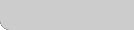About Us  |  Help  |  Register  |  Privacy Policy  |  Membership Agreement